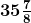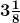# A skirt that is

Question:

A skirt that iscm long has a hem ofcm. How long will the skirt be if the hem is let down?

Solution:

From the question it is given that,

Length of the skirt =cm = 287/8 cm

Dimension of hem =cm = 25/8 cm

Length of skirt, if hem is let down = ((287/8) + (25/8)) cm

= 312/8 cm

= 39 cm